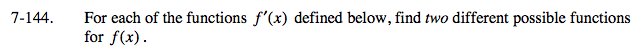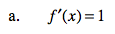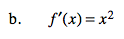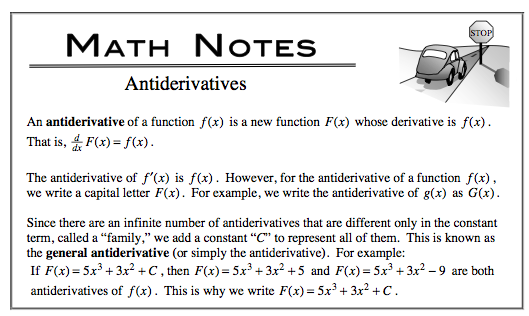### Home > CALC > Chapter 7 > Lesson 7.3.4 > Problem7-144

7-144.
1. For each of the functions f ′ (x) defined below, find two different possible functions for f(x). Homework Help ✎

1. f ′(x) = 1

2. f ′(x) = x2